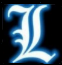2020-2-19 6433

## 背景## 正文### 一、识别图片```def main(counts, captcha_image_file):
# 结果输出dir
OUTPUT_FOLDER = './result'
# 基础数据dir

# 加载图像并将其转换成灰度级
gray = cv2.cvtColor(image, cv2.COLOR_BGR2GRAY)

# 在图像周围添加一些填充物
gray = cv2.copyMakeBorder(gray, 1, 1, 1, 1, cv2.BORDER_REPLICATE)

# 阈值图像（转换为纯黑色和白色）
# threshold:    输入图像,阈值,输出图像的最大值,阈值的类型,目标图像
# 转换第一次，有浅色情况所以下面转第二次
thresh = cv2.threshold(gray, 200, 255, cv2.THRESH_TOZERO)
# 将图片转为黑白
thresh = cv2.threshold(thresh, 0, 255, cv2.THRESH_BINARY_INV | cv2.THRESH_OTSU)

# 找到图像的轮廓（连续像素块）
contours = cv2.findContours(thresh.copy(), cv2.RETR_EXTERNAL, cv2.CHAIN_APPROX_SIMPLE)

# OpenCV版本适应
contours = contours if imutils.is_cv2() else contours
letter_image_regions = []

# 遍历轮廓，并提取字母
for contour in contours:
# 获取包含轮廓的矩形
(x, y, w, h) = cv2.boundingRect(contour)

# 比较轮廓的宽度和高度来检测字母
if w / h > 1.25:
# 轮廓太宽,把它分成两个字母区域
half_width = int(w / 2)
letter_image_regions.append((x, y, half_width, h))
letter_image_regions.append((x + half_width, y, half_width, h))
else:
# 正常字母
letter_image_regions.append((x, y, w, h))

# 根据X坐标对检测到的字母图像进行排序，以确保我们从左到右处理它们，因此我们将正确的图像与正确的字母匹配。
letter_image_regions = sorted(letter_image_regions, key=lambda x: x)

# Save image
retStr = ''
nCnt = 0
for letter_bounding_box, letter_text in zip(letter_image_regions, captcha_correct_text):
nCnt += 1
# 抓取图像中字母的坐标
x, y, w, h = letter_bounding_box
# 从原始图像中提取具有边缘的2像素边缘的字母
letter_image = gray[y - 2:y + h + 2, x - 2:x + w + 2]

# 获取文件夹以保存图像
save_path = os.path.join(OUTPUT_FOLDER, letter_text)
# 如果输出目录不存在，则创建它
if not os.path.exists(save_path):
os.makedirs(save_path)

# 将字母图像写入文件
count = counts.get(letter_text, 1)
p = os.path.join(save_path, "{}.png".format(str(count).zfill(6)))
retStr += letter_text
cv2.imwrite(p, letter_image)

counts[letter_text] = count + 1

return (retStr)```

```dicT = {}
a = list(a)
try:
# 这里做个打印
if len(a)>=3:
if a == 'x':
print(a+'*'+a+'='+str((int(a)*int(a))))
else:
print(a+'+'+a+'='+str((int(a)+int(a))))
except:
raise```### 二、训练模型

Keras 是一个用 Python 编写的高级神经网络 API，它能够以 TensorFlow, CNTK, 或者 Theano 作为后端运行。Keras 的开发重点是支持快速的实验。能够以最小的时延把你的想法转换为实验结果，是做好研究的关键。 如果你在以下情况下需要深度学习库，请使用 Keras：

• 允许简单而快速的原型设计（由于用户友好，高度模块化，可扩展性）。
• 同时支持卷积神经网络和循环神经网络，以及两者的组合。
• 在 CPU 和 GPU 上无缝运行

```LETTER_IMAGES_FOLDER = "result"
MODEL_LABELS_FILENAME = "model_labels.dat"

# 初始化
data = []
labels = []

# 循环result中的图片
for image_file in paths.list_images(LETTER_IMAGES_FOLDER):
# 读取图片转灰度
image = cv2.cvtColor(image, cv2.COLOR_BGR2GRAY)
# 重置图片大小，统一为20x20 pixel
image = resize_to_fit(image, 20, 20)
# 在原image数组的3位置添加数据，是为了给keras用
image = np.expand_dims(image, axis=2)
# 获取文件名
label = image_file.split(os.path.sep)[-2]
# 加入到数组以备后续训练模型
data.append(image)
labels.append(label)

# 下述模型训练的代码未做改动
data = np.array(data, dtype="float") / 255.0
labels = np.array(labels)
(X_train, X_test, Y_train, Y_test) = train_test_split(data, labels, test_size=0.25, random_state=0)
lb = LabelBinarizer().fit(Y_train)
Y_train = lb.transform(Y_train)
Y_test = lb.transform(Y_test)
# one-hot编码保存映射文件
with open(MODEL_LABELS_FILENAME, "wb") as f:
pickle.dump(lb, f)

model = Sequential()

model.fit(X_train, Y_train, validation_data=(X_test, Y_test), batch_size=13, epochs=10, verbose=1)

model.save(MODEL_FILENAME)```### 三、开始验证

```MODEL_FILENAME = "captcha_model.hdf5"
MODEL_LABELS_FILENAME = "model_labels.dat"
# 要破解的图片文件夹所在目录

# 载入映射文件和模型
with open(MODEL_LABELS_FILENAME, "rb") as f:

```captcha_image_files = list(paths.list_images(CAPTCHA_IMAGE_FOLDER))
# loop over the image paths
# Load the image and convert it to grayscale
image = cv2.cvtColor(image, cv2.COLOR_BGR2GRAY)
image = cv2.copyMakeBorder(image, 1, 1, 1, 1, cv2.BORDER_REPLICATE)
# 转换第一次，有浅色情况所以下面转第二次
thresh = cv2.threshold(image, 200, 255, cv2.THRESH_TOZERO)
# 将图片转为黑白
thresh = cv2.threshold(thresh, 0, 255, cv2.THRESH_BINARY_INV | cv2.THRESH_OTSU)
# 找到图像的轮廓（连续像素块）
contours = cv2.findContours(thresh.copy(), cv2.RETR_EXTERNAL, cv2.CHAIN_APPROX_SIMPLE)
# OpenCV版本适应
contours = contours if imutils.is_cv2() else contours
# 遍历四个轮廓中的每一个并提取每个轮廓中的字母，这里封装统一函数，不作显示，详见example
letter_image_regions = contours_regions(contrours)
# 输出demo,做轮廓的标记
output = cv2.merge([image] * 3)
predictions = []
for letter_bounding_box in letter_image_regions:
x, y, w, h = letter_bounding_box
letter_image = image[y - 2:y + h + 2, x - 2:x + w + 2]
# 同样的resize
letter_image = resize_to_fit(letter_image, 20, 20)
letter_image = np.expand_dims(letter_image, axis=2)
letter_image = np.expand_dims(letter_image, axis=0)
prediction = model.predict(letter_image)
letter = lb.inverse_transform(prediction)
predictions.append(letter)
cv2.rectangle(output, (x - 2, y - 2), (x + w + 4, y + h + 4), (0, 255, 0), 1)
cv2.putText(output, letter, (x - 5, y - 5), cv2.FONT_HERSHEY_SIMPLEX, 0.55, (0, 255, 0), 2)

# 至此predictions中即为破解解析后的各个字符的list了，接下来是一些便利性的打印，不作展示
print(predictions)```## 总结

• 通过cv2读入并转换图片，这部分在blog中有不少示例了，所以用的相对熟练。这也是能通过示例example的黑白字母验证码引申到彩色的算式验证码上的原因所在；
• keras是第一次使用，基本上是照搬的示例了，用的还比较顺手。虽不是第一次训练model，但也是第一次这么流程清晰的使用神经网络；